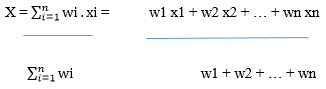код Weighted Average Calculator - Calculatorology

## Weighted Average Calculator

 Weight Data number Weighted average: Calculation:

### Weighted Average Calculator

This calculator is used to determine the weighted average of a set of data. It has two columns where you enter the values of weight and Data number. The weighted average calculator has three control buttons. The ‘Calculate’ button is used to execute the calculations after filling in the values in their respective text fields. If you want to calculate new values, you will use the ‘Reset’ button. It is the quickest way of erasing the previous calculations from the text fields. In situations where you have a large set of data, you click on the ‘Add row’ button. It is used to add single rows depending on the number of values entered in the text fields.

##### For example;
If the weight measurements are 40, 45, 60, 72, 76, 80 and the data number; 1, 2, 3,4,5,6, determine the weighted average.

##### Solution
You will enter the weight measurements in the first column and the data numbers in the second column respectively. Click on the ‘Calculate’ button to initiate the conversion.

The results will be displayed as;

Weighted Average = 3.90884718

Calculation;

(40×1+45×2+60×3+72×4+76×5+80×6) / (40+45+60+72+76+80) = 3.90884718

##### Formula of calculating the weighted average
This calculator uses a particular formula in executing the calculations. It makes it easier to determine a large set of values as it gives accurate results within a shorter period.It means that the weighted average (x) is calculated by summing up the products of the weight by the data number which is then divided by the sum of the weights. This calculator can be used to determine the weighted average of multiple values making it useful in executing conversions of large data. If you have a new set of data, you can use the ‘Reset’ button as it will clear all the text fields with a single click.